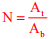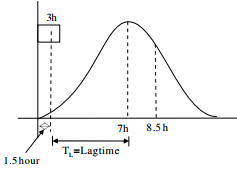# Environmental Engineering - Online Test

Q1. Which one of the following is NOT present in the acid rain?
Explaination / Solution:
No Explaination.

Q2. The bacteria in milk are destroyed when it _________ heated to 80 degree Celsius.
Explaination / Solution:
No Explaination.

Q3. Pre-cursors to photochemical oxidants are
Explaination / Solution:
No Explaination.

Q4. It was decided to construct a fabric filter, using bags of 0.45 m diameter and 7.5 m long, for removing industrial stack gas containing particulates. The expected rate of airflow into the filter is 10m3 /s. If the filtering velocity is 2.0 m/min, the minimum number of bags (rounded to nearest higher integer) required for continuous cleaning operation is
Explaination / Solution:

Given, D=0.45m, L=7.5m
No. of fabric filter bags,Total area of filter (Af) = (10 × 60)/2 = 300m2
Area of one bag (Ab) = πdL = π×0.45×7.5 = 10.60m2
N = 300/10.60 = 28.28 ~ 29

Q5. Match the items in Group – I with those in Group – II and choose the right combination.
Group - I                                                  Group - II
P. Activated sludge process                    1. Nitrifiers and denitrifiers
Q. Rising of sludge                                  2. Autotrophic bacteria
R. Conventional nitrification                    3. Heterotrophic bacteria
S. Biological nitrogen removal                4. Denitrifiers
Explaination / Solution:
No Explaination.

Q6. SO2 and CO adversely affect
Explaination / Solution:

Carbon monoxide effects the bloods carrying capacity

Q7. An isolated 3-h rainfall event on a small catchment produces a hydrograph peak and point of inflection on the falling limb of the hydrograph at 7 hours and 8.5 hours respectively, after the start of the rainfall. Assuming, no losses and no base flow contribution, the time of concentration (in hours) for this catchment is approximately
Explaination / Solution:For small catchment, time of concentration is equal to lag time of peak flow.
Tc =7−1.5=5.5h

Q8. The Muskingum model of routing a flood through a stream reach is expressed as O2 = K0I2 + K1I1 + K2O1, where K0, K1 and K2 are the routing coefficients for the concerned reach, I1 and I2 are the inflows to reach, and O1 and O2 are the are the outflows from the reach corresponding to time steps 1 and 2 respectively. The sum of K0, K1 and K2 of the model is
Explaination / Solution:
No Explaination.

Q9. The dominating microorganisms in an activated sludge process reactor are
Explaination / Solution:
No Explaination.

Q10. The two air pollution control devices that are usually used to remove very fine particles from the flue gas are
Explaination / Solution:
No Explaination.